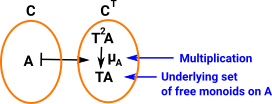# Maths - Category Theory - Adjunctions

### Example - Monoid

'term' or 'word' algebras determines an adjunction

This example looks at extremes such as the forgetful functor in one direction (which removes all structure and leaves the underlying set) and the free algebra in the other direction (see Presentation of a group. Free algebra has generators without constraints).

### Free Monoid

Here we have a set {a,b,c...} with a binary operation. When we apply the binary operation to two elements of the set, say a × b, we get a new element which we could notate as 'ab'.

### List

Another way to think of this free monoid is by representing a × b = [a,b]

So this list is an element of the free monoid. The function which takes a list 'T A' to the new element 'A' is called θ:

θ : T A -> A

Here think of 'T' as a generic notation for 'List'.

### Functors between Set and MonoidSo,

• G is the forgetful functor which goes from monoid to set and removes the structure (multiplication and identity) and,
• F goes from set to monoid and creates a free monoid, since there are no relations, the elements of the set don't interact and remain as a word or list.

therefore:

• μ : 1monoid => FG is a transformation within monoid, which forgets the existing structure and creates a free structure.
• ξ : GF => 1set is a transformation within set, which creates a free monoid (list) and then forgets the structure to return the elements of the set.

#### Forgetful functor from monoid to set.

Takes the algebra and returns the underlying set#### Free functor from set to monoid.

Takes the underlying set and returns a monoid with a multiplication.So we have two functors 'F' and 'G'. If we start with set and apply the free functor 'F' first and then the forgetful functor 'G' which we denote as 'GF' we get a set containing all possible lists of elements which is 'T'.

The final and initial adjunctions from 'T' are given by the base category (in this case set) with the monoid which can be generated in the following ways:

#### Eilenberg-Moore Category for Monoid

This is final in the categories for the monad.

• The objects are θ : T A -> A
• The morphisms are the same as the underlying set: A->B.

So we just 'freely' apply θ to the elements of the underlying set to produce the elements of the monoid.

#### Kleisli Category for Monoid

This is initial in the categories for the monad.

• The objects are the same as the underlying set: A.
• The morphisms are: A -> T B.

In other words the morphisms wrap the elements in a list: A -> List B.

This makes function composition more complicated. When we compose

A -> List B

with B -> List C

we get

A -> List (List C)

but we want:

A -> List C

So we have to apply μ to get from List (List C) to List C.

In this case μ just flattens the nested list to produce one list with all the elements.

### Other Pages on this site

• Category of graphs described on page here.
• Implementing Graphs in FriCas program is discussed on page here.
• Implementing Posets in FriCas program is discussed on page here.

Where I can, I have put links to Amazon for books that are relevant to the subject, click on the appropriate country flag to get more details of the book or to buy it from them.Conceptual Mathematics - This is a book about category theory that does not assume an extensive knowledge over a wide area of mathematics. The style of the book is a bit quirky though.